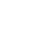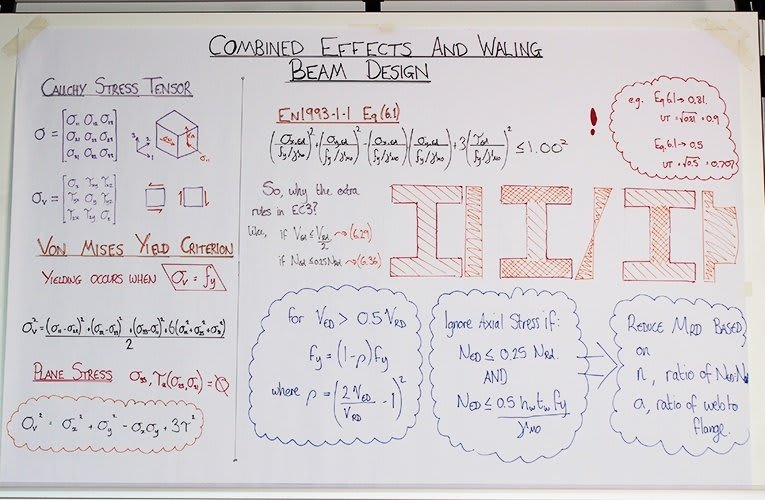# Combined Effects and Waling Beam Design

03 MayWelcome back to the drawing board and today are we talking about the design of walers as well as the combined effects that act upon them.  When I say combined effects I’m talking about axial force, bending moment and shear forces all acting on the waler at the same time. I won’t be talking about torsion because you don't usually see torsional effects on the kind of walers that we do.

### Transcription

I’m going to start by mentioning the cauchy stress tensor, you don't have to understand all of it but it's just a way of expressing the states of stress at any point in the material by combining this nine part matrix which includes any forces in any direction acting on any plane.

These three guys down the middle are the direct stresses so the compression in each direction. And these here, you can pair them up, these are the (shear) stresses that are going around in each plane. The (shear) stresses going around anti-clockwise will have to be equaled by the (shear) stresses going clockwise in order to maintain balance.

So these two, these two and these two can be paired up. It makes writing this next equation quite a lot simpler. Von Mises Yield Criterion is basically the stress that you get here and it has to be less than the yield strength of the material. If it isn’t then material begins to yield plastically, if it is then your material will stay put and that’s fine.

So writing out this equation you have subtraction of the main (principal) stresses all squared and then you have lots of your shear stresses and that’s all divided by two.

So I was talking about subtracting the principal stresses and I missed one of the most interesting facts about the mises criterion. If you have equal stresses in all principal planes it will be almost the same as having no stresses at all. If you squash something from say two directions, the only place it could deform is by squidgy out up and down. But, if you're also applying the stress there it can’t move anywhere and there can be no yield if there is no definition. So what’s quite interesting is if you actually apply an equal force to one plane and an equal force to the other plane it’s almost the same as having this scenario but with these forces taken away, which basically equates to a tension force equal to a single one of these principal stresses. Not very useful but very interesting.

I'm not going to go into it too deeply but what you can actually get is if you read the Eurocode there is a very similar equation that’s the very first equation that you have at the start of the Euroode section 6, in Eurocode 3.

Instead of doing the square of all of these different stresses it takes the utilization in each of these stress directions (stress planes) and then it compares that and as long as that utilization all together is less than one then you haven’t over utilized the steel.

One really important thing to mention is that if you come out this equation and you have a utilization of 0.81 that’s not 81%, it’s 90% because although the square root of one is one, you still have to square root because that is the square there.

So if you have 50% coming out of here of 0.5, you’re actually 70% utilized so if you did get the answer of 0.5 don’t think to yourself “oh I could double the load on that,” you couldn’t, you could only add an extra 30%.

Why then are there all these extra rules in EC three. Because the Von Mises Yield Criterion makes you think that if I got a point of stress that was bigger than my yield stress then my thing would start bending. Well that is assuming an elastic distribution and if you were dealing with a class three or a class four section then that would certainly be true. But, most of what we deal with in steel, certainly most of the UKB and UKC sections, are class one or class two and you can use plastic redistribution of stresses. This doesn’t just apply in redistributed moments along the length of the beam this also applies within the structure of the beam itself.

If you look carefully at them, the axial load this will usually be uniformly distributed throughout the depth of the section. Bending moments, bigger at the sides, if you’re talking elastic, and then not so much in the middle. And then shear forces generally speaking high in the middle and not so much at the sides. So it gives you these extra rules so that you can redistribute, move around the stresses and get every single part more utilized rather than over utilizing one part of it.

So taking a bit of a closer look. So if your shear force is less than half of your shear existence then you don’t even have to worry about it because this amount used here isn't significant enough that it would affect these two. If it is then it’s not a linear relationship, it’s not like if I’ve used 80% of my shear I only have 20% strength in my beam left. You get to use this nifty little rule here, which gives you this “Rho” factor. You reduce the effective yield strength of the material based on how much of the shear strength you’ve utilized.

You can ignore axial stress as well if that's not very high, again the reason being that if you’ve only used a quarter of the strength of the material think about triangles only taking up half of the area. It's that similar principle is that if you’ve only used a little bit of this then you could this could easily knock off the end of that triangle and redistribute some of that force and some of that stress into this web here.

And if you do have an axial force that is greater, then you reduce the bending moment and this equation is difference for every single beam. They all follow a ratio of much of your (correction: axial force compared to your axial resistance) and a ratio of how much web to how much flange. Or in the case of a Square hollow section for instance how much you’ve got on the top and bottom compared to the two sides if it was a square section.

But for the case of the I sections, the UCs and the UB’s you take a ratio of force to resistance, you take a ratio of how much web to flange and you plug those into the equations from the Eurocode and it gives you a slight reduction. Just because you’ve used half of your axial strength doesn’t mean you’re going to have to reduce your bending resistance by half, again it lets you move things around and gives you a less conservative but a much more economical strength out of your beam.

I haven’t covered everything but hopefully that gives you a little bit of instruction in the combined effects to EC3.

Steven Lloyd